Your browser (Unknown 0) is no longer supported. Some parts of the website may not work correctly. Please update your browser.

#### PrettyTiling

Given a sequence of squares, find the minimum number of rotations needed to obtain a pretty sequence.

Tom is decorating the pavement in his garden with N square tiles. Each tile is divided into four triangles of different colors (white - 'W', red - 'R', green - 'G' and blue - 'B'). A tile is described as a string of four characters denoting respectively, the color of the upper, right, bottom and left triangle. For example, the tile in the figure below is described as "WRGB".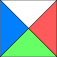Tom arranged the tiles in a row and decided to rotate some of them to obtain a pretty sequence. He considers a sequence of tiles pretty if each pair of adjacent tiles shares one side of the same color.

Write a function:

class Solution { public int solution(String[] A); }

that, given an array A of N strings, representing the sequence of tiles, returns the minimum number of 90-degree rotations (clockwise or counter-clockwise) that Tom has to perform.

Examples:

1. Given A = ["RGBW", "GBRW"], the function should return 1.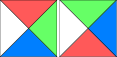Tom can rotate the second tile counter-clockwise once to obtain a pretty sequence.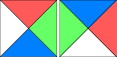2. Given A = ["WBGR", "WBGR", "WRGB", "WRGB", "RBGW"], the function should return 4.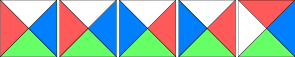Tom can obtain a pretty sequence by rotating the first and third tiles counter-clockwise and the second and fourth tiles clockwise.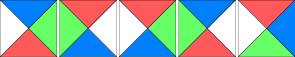3. Given A = ["RBGW", "GBRW", "RWGB", "GBRW"], the function should return 2.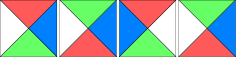Tom can rotate the first tile clockwise twice to obtain a pretty sequence.4. Given A = ["GBRW", "RBGW", "BWGR", "BRGW"], the function should return 2.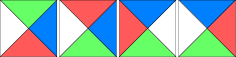Tom can rotate the first two tiles clockwise to obtain a pretty sequence.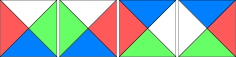Write an efficient algorithm for the following assumptions:

• N is an integer within the range [1..100,000];
• string representing a tile has 4 letters (exactly one occurrence of 'R', 'G', 'B' and 'W').